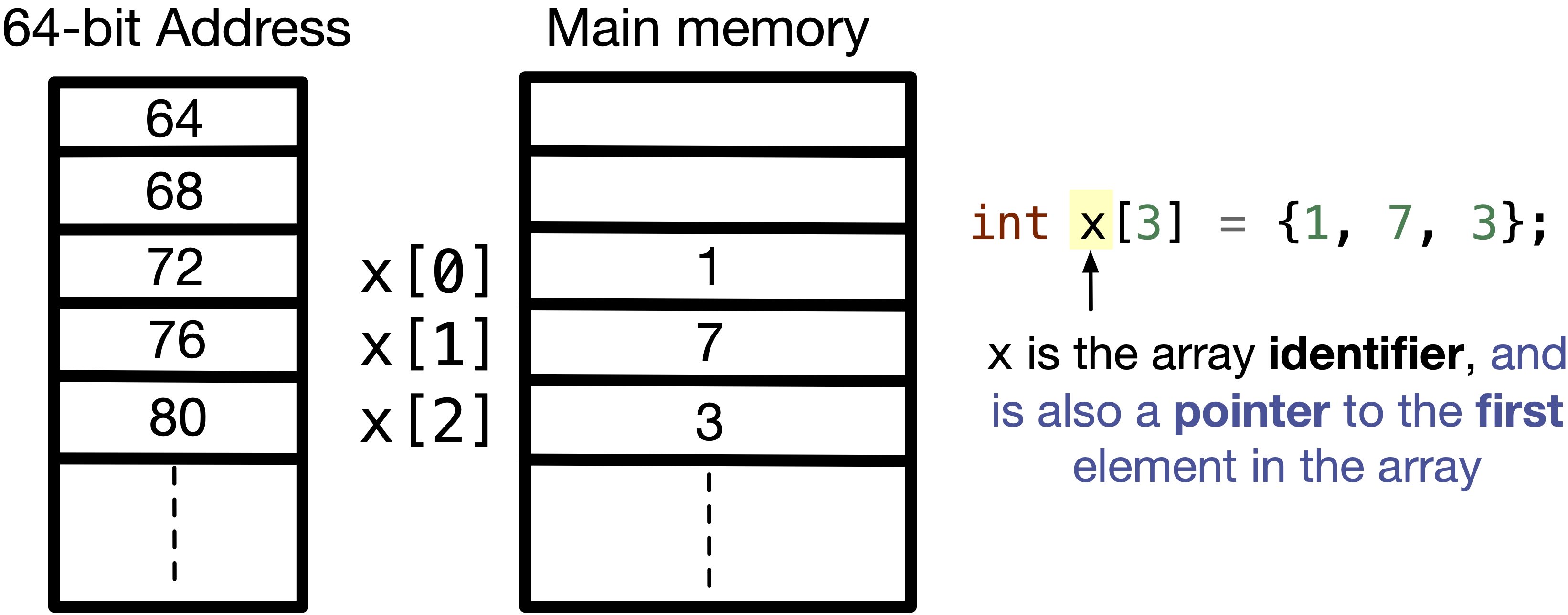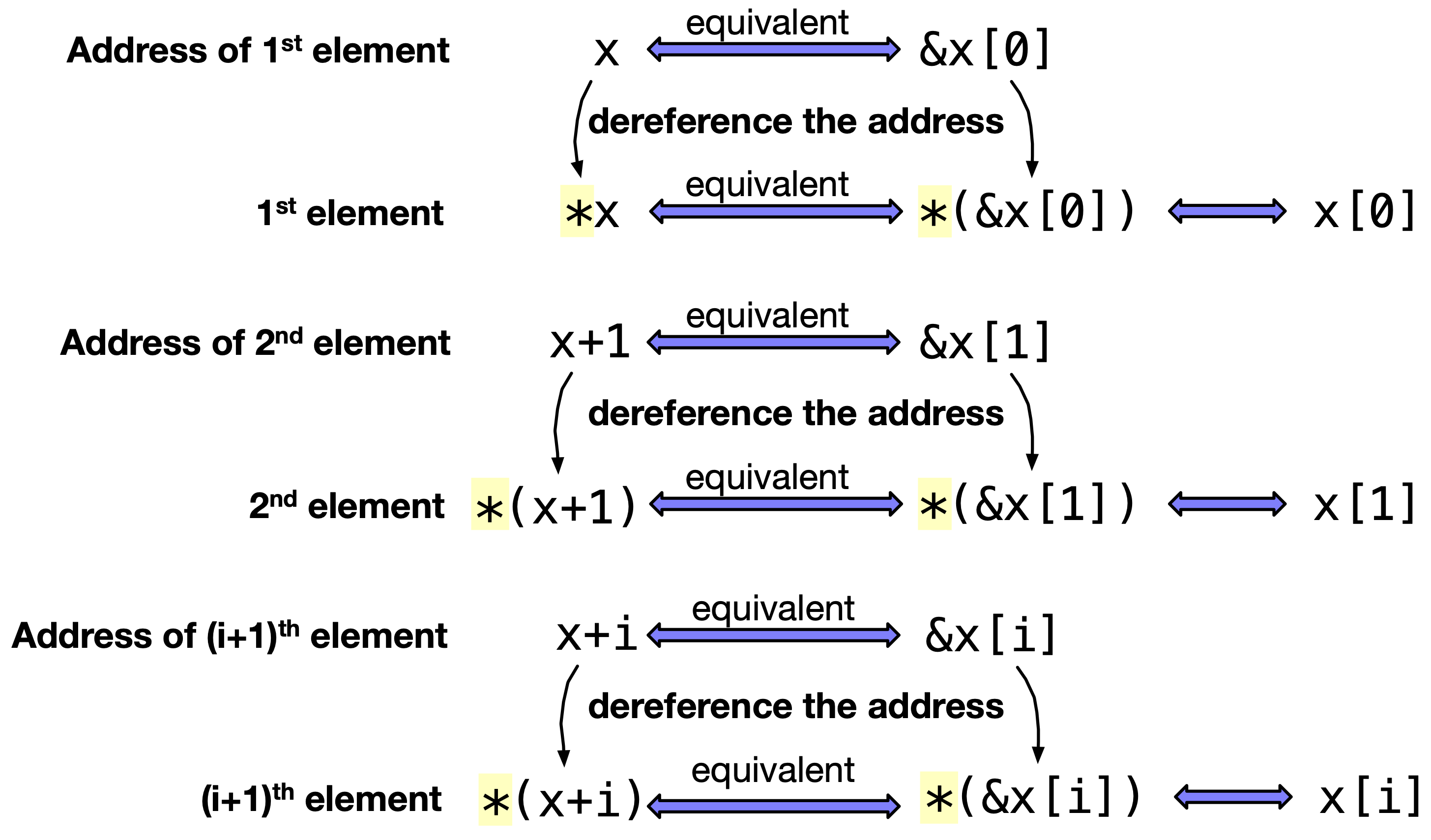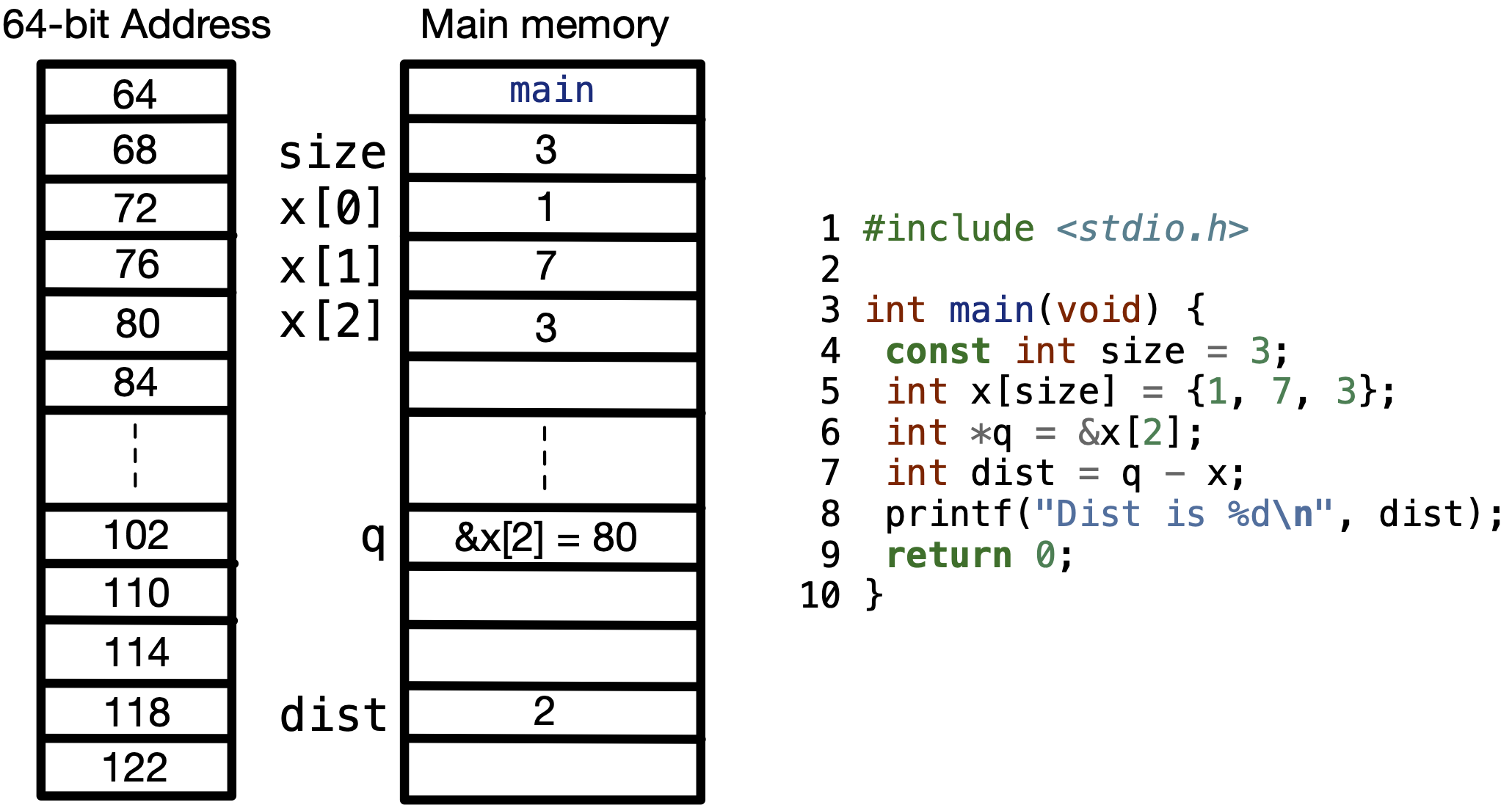# 7.2. What are arrays, and how are they stored?#

When you declare an array using the C programming language, all the elements in the array are stored contiguously in the main memory.

For example, in the following figure, an array of $$3$$ elements is stored in the main memory. We can see the addresses of each element is separated by $$4$$, while we claim that variables are stored contiguously (one after the other). This is because a int variable is stored using $$4$$ bytes. Hence, if x is stored at address $$72$$, it will take address $$73$$, $$74$$ and $$75$$ to store the contents of x. Then, x will start at address $$76$$.Fig. 7.5 Array elements are stored contiguously in a the main memory. Since the array is byte addressable, if the address of the first element is $$72$$, the address of the second element is $$4$$ bytes after that at $$76$$. Why $$4$$ bytes? This is because a int variable is stored in $$4$$ bytes, and if variables will be contiguous, their addresses will be separated by $$4$$ bytes.#

In the figure above, we also say that x is not just the identifier of the array, instead it is also a pointer to the first element in the array. This means that x is equivalent to &x. Hence, if we dereference x, we get the first element of the array *x $$\rightarrow$$ *(&x) $$\rightarrow$$ x.

We can refer to the second element in the array, if we add $$1$$ to the address of the first element x, i.e. x+1 is equivalent to x.

The following figure summarizes how can x be used as a pointer to different elements in the array.Fig. 7.6 x is the identifier of the array, but it can also be used as a pointer to the first element in the array.#

Given that x is a pointer to the first element in the array, we can pass this pointer to a function, and be able to access elements in the array!

## 7.2.1. Pointer Arithmetic#

Given that we now understand that an array identifier is also a pointer to the first element in an array. Within the context of arrays, pointer arithmetic plays an important role in understanding how elements in an array are contiguously stored in the main memory.

For example, if I have an array of $$3$$ elements, int x[] = {1, 7, 3};, the addresses in the main memory will look something like in the following figure.Fig. 7.7 If we get the difference between two addresses of two elements, the result is the difference between the addresses divided by sizeof() stored in the address. For example, dist = (80 - 72) / sizeof(int) $$\rightarrow$$ dist = 8 / 4 = 2.#

In the above figure, we have in line 6 int *q = &x;. So q is holding the address of x, which is $$80$$.

In line 7, int dist = q - x;, where x is also the address of x. Hence, dist = &x - &x. However, we don’t just get the difference between the two addresses. Instead, we divide the result by sizeof(int), which is the size of the data stored at these two addresses. Given that, dist = (80 - 72) / sizeof(int) $$\rightarrow$$ dist = 2.

## 7.2.2. Practice Problem#

The following question was question 5 in Winter 2022 midterm exam for APS 105 in the University of Toronto. The question requires that you print the output of the following program.

Code


#include <stdio.h>
int main(void) {
int first = 1, second = 2, data = {10, 20, 30, 40};
int *third = &second, *fourth = &first, *fifth = data + first + 1;
(*third)++;
(*fourth)++;
data[second] = *fifth + first + *third + *fourth;
printf("first = %d, second = %d, third = %d, fourth = %d, fifth = %d\n",
first, second, *third, *fourth, *fifth);
for (int i = 0; i < 4; i++) {
printf("%d, ", data[i]);
}
printf("\n");
return 0;
}



In line $$3$$, first = 1, second = 2, data = 10, data = 20, data = 30, data = 40.

In line $$4$$, third = &second, fourth = &first, fifth = data + first + 1 = &data + first + 1 = &data + 2 = &data. Here, &data + 2 = &data because adding $$2$$ to the address of data adds $$2 \times$$ sizeof(int), which is the address of data.

In line $$5$$, (*third)++ $$\rightarrow$$ second++, so second = 3.

In line $$6$$, (*fourth)++ $$\rightarrow$$ first++, so first = 2.

In line $$7$$, data[second] = *fifth + first + *third + *fourth = data + 2 + 3 + 2 = 37. Hence, data = 37.

In line $$8$$, we print first = 2, second = 3, third = 3, fourth = 2, fifth = 30.

In line $$11$$, we print 10, 20, 30, 37,.

The output is

first = 2, second = 3, third = 3, fourth = 2, fifth = 30
10, 20, 30, 37,


0 Questions

X